Classical Mechanics

# Moment of Inertia of Point Masses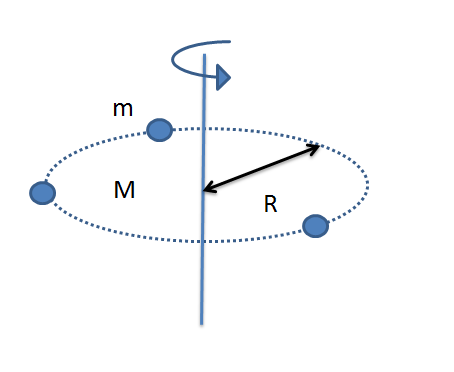A ring of mass $M = 3 \text{ kg}$ and radius $R = 3 \text{ m}$ rotates about its center. If three identical balls each of mass $m = 6 \text{ kg}$ are attached on the ring, what is the total moment of inertia of the ring and balls?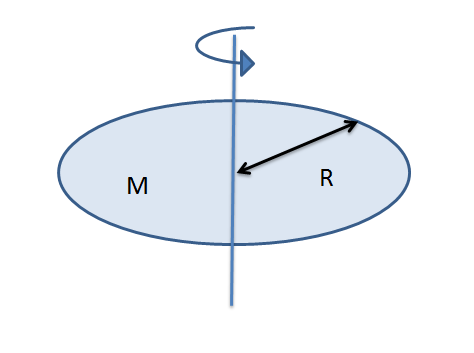A disk of mass $M = 2 \text{ kg}$ and radius $R = 6 \text{ m}$ rotates about its center. Find its moment of inertia.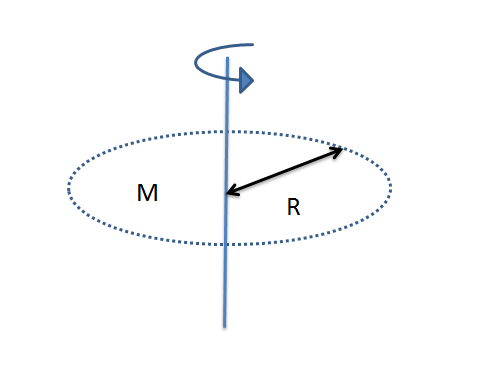A ring of mass $M = 8 \text{ kg}$ and radius $R = 7 \text{ m}$ rotates about its center. Find its moment of inertia.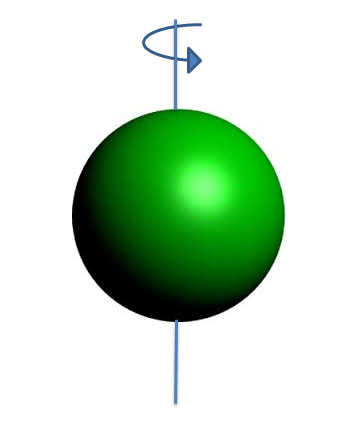A solid sphere of mass $M = 15 \text{ kg}$ with homogeneous density rotates about its center. If the sphere's radius is $R=5\text{ m},$ what is its moment of inertia?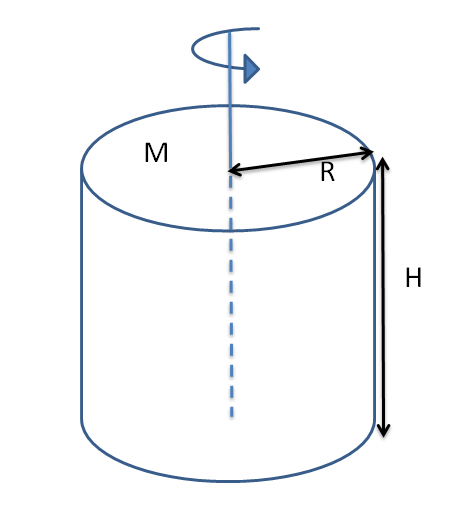A solid cylinder of mass $M = 8 \text{ kg}$ with homogeneous density has a circular base of radius $R = 5 \text{ m}$ and a height of $H = 5 \text{ m}.$ If the cylinder rotates about its center, as illustrated in the above diagram, what is its moment of inertia?

×